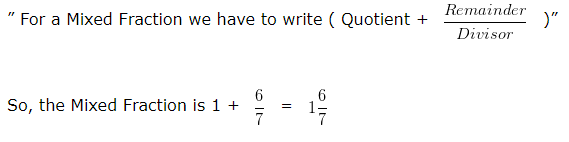# Convert Improper Fraction to Mixed Fraction

To Convert Improper Fraction to Mixed Fraction, we follow the steps given below:-

Step I: Divide the Numerator by the Denominator.

Step II: Write the Mixed Fraction as: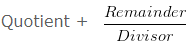### Convert Improper Fraction to Mixed Fraction

Question 1

Convert 3/2 into Mixed Fraction.

Explanation

Given Fraction:

3/2

Divide the Numerator by the Denominator

On dividing 3 by 2

We get Quotient = 1

Remainder = 1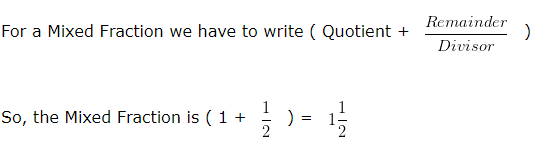Question 2

Convert 5/3 into Mixed Fraction.

Explanation

Given Fraction:

5/3

Divide the Numerator by the Denominator

On dividing 5 by 3

We get Quotient = 1

Remainder = 2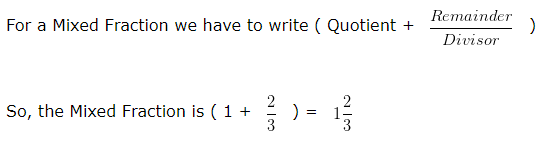Question 3

Convert 12/7 into Mixed Fraction.

Explanation

Given Fraction:

12/7

Divide the Numerator by the Denominator

On dividing 12 by 7

We get Quotient = 1

Remainder = 5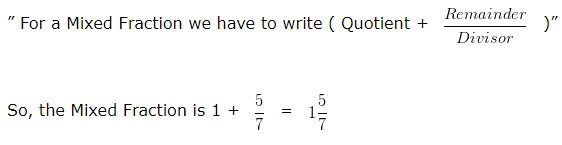Question 4

Convert 5/4 into Mixed Fraction.

Explanation

Given Fraction:

5/4

Divide the Numerator by the Denominator

On dividing 5 by 4

We get Quotient = 1

Remainder = 1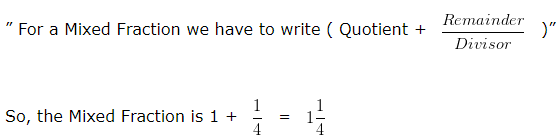Question 5

Convert 13/7 into Mixed Fraction.

Explanation

Given Fraction:

13/7

Divide the Numerator by the Denominator

On dividing 13 by 7

We get Quotient = 1

Remainder = 6